StatLect

# Statistical model

A statistical model is a set of assumptions about the probability distribution that generated some observed data.## Examples

We provide here some examples of statistical models.

Example Suppose that we randomly drawindividuals from a certain population and measure their height. The measurements can be regarded as realizations ofrandom variables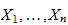. In principle, these random variables could have any probability distribution. If we assume that they have a normal distribution, as it is often done for height measurements, then we are formulating a statistical model: we are placing a restriction on the set of probability distributions that could have generated the data.

Example In the previous example, the random variablescould have some form of dependence. If we assume that they are statistically independent, then we are placing a further restriction on their joint distribution, that is, we are adding an assumption to our statistical model.

Example Suppose that for the sameindividuals we also collect weight measurements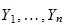, and we assume that there is a linear relation between weight and height, described by a regression equation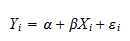whereandare regression coefficients andis an error term. This is a statistical model because we have placed a restriction on the set of joint distributions that could have generated the couples: we have ruled out all the joint distributions in which the two variables have a relation that cannot be described by the regression equation.

Example If we assume that all the errorsin the previous regression equation have the same variance (i.e., the errors are not heteroskedastic), then we are placing a further restriction on the set of data-generating distributions. Thus, we have yet another statistical model.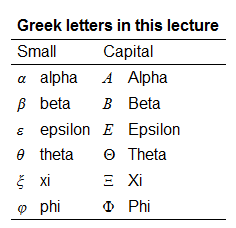## Formal definition

As shown in the previous examples, a model is a set of probability distributions that might have generated the data sample.

The sample, denoted by, is a vector of data. It can be thought of as the realization of a random vector.

In principle,could have any joint probability distribution.

If we assume that the distribution ofbelongs to a certain set of distributions, thenis called a statistical model (see, e.g., McCullagh 2002).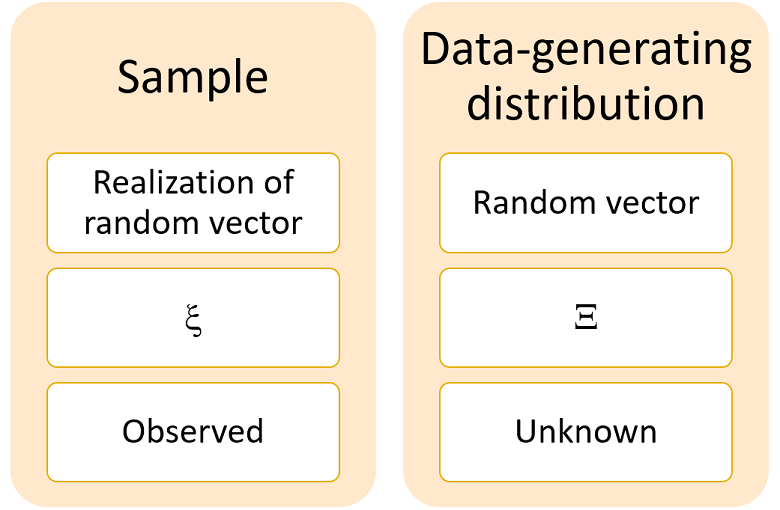## Parametric model

When the statistical modelis put into correspondence with a setof real vectors, then we have a parametric model.

The setis called parameter space and any one of its membersis called a parameter.

Example Assume, as we did in the first example above, that the height measurementscome from a normal distribution. Then,is the set of all normal distributions. But a normal distribution is completely characterized by its meanand its variance. As a consequence, each member ofis put in correspondence with a vector of parameters. The meancan take any real value and the varianceneeds to be positive. Therefore, the parameter space is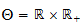.

## Nonparametric model

When a correspondence betweenand a parameter space is not specified, then we have a nonparametric model.

In this case, we use techniques that allow us to directly analyze, for example:

1. multivariate kernel density estimation (the distribution of the data is recovered through histogram-like estimators);

2. kernel regression (the joint distribution estimated with kernel density methods is used to derive the distribution of some variables conditional on others).

These models, used in nonparametric statistics, make minimal assumptions about the data-generating distribution. They allow the data to "speak for themselves" (e.g., Hazelton 2015).

## How is a statistical model used?

What do we do after formulating a parametric statistical model?

The typical things we do are:

1. parameter estimation: we produce a guess of the parameterassociated to the true distribution (the one that generated the data); the guess is produced using so-called estimation methods, such as:

2. set estimation: we search for a small subset ofthat contains the true parameterwith high probability;

3. hypothesis testing: we place further restrictions on the set of possible data-generating distributions; then, we test whether the restrictions are supported by the data;

4. Bayesian updating: we first assign a prior distribution to the parameters; then we use the sample data to update the distribution.

## Conditional vs unconditional models

In conditional models (also called discriminative models), the sample is partitioned into input and output data, as in the regression example above. The statistical model is obtained by placing some restrictions on the conditional probability distribution of the outputs given the inputs.

This is in contrast to unconditional models (also called generative models), used to analyze the joint distribution of inputs and outputs.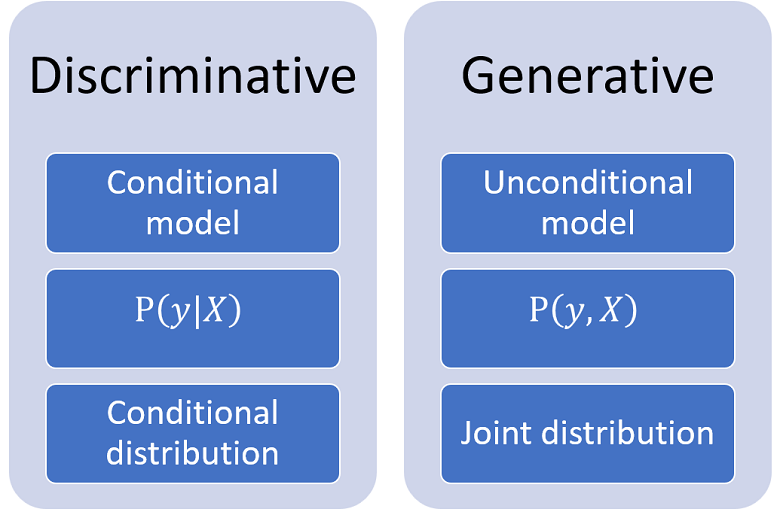## Regression vs classification

There are two classes of conditional models:

1. regression models, in which the output variable is continuous; for example:

1. the linear regression model, which postulates the existence of a linear relation between the outputs (dependent variables) and the inputs (explanatory variables);

2. non-linear regression, in which the input-output mapping can be non-linear.

2. classification models, in which the output variable is discrete (or categorical); for example:

1. the logistic classification model (or logit model), used to model the influence of some explanatory variables on a binary outcome;

2. the multinomial logit, in which the response variable can take more than two discrete values.

Understanding the distinction between regression and classification is essential for a correct choice of a statistical model.

## Predictive and machine-learning models

Conditional statistical models can be used to make predictions of unseen outputs given observed inputs.

There are models that also allow us to make such predictions, but without specifying a set of conditional probability distributions (not even implicitly). Strictly speaking, they are not statistical models. They can be broadly classified as predictive models.

Predictive models can be seen as algorithms that try to accurately reproduce a mapping between inputs and outputs (see, e.g., Breiman 2001).

Several models used in the machine learning field belong to the class of predictive models. For example:

## Parsimonious models

A fundamental characteristic of a parametric statistical model is the dimension of its parameter space, which is equal to the number of entries of the parameter vectors.

Example The dimension of a linear regression model is equal to the number of regression coefficients, which in turn is equal to the number of input variables.

Models that have a large dimension are often difficult to estimate, as the estimators of the parameter vector tend to have high variance.

Moreover, large models are prone to over-fitting: they tend to accurately fit the sample data, and to poorly predict out-of-sample data.

For these reasons, we often try to specify parsimonious statistical models, that is, simple models with few parameters. Despite its simplicity, a parsimonious model should be able to reproduce all the main characteristics of the data in a satisfactory manner.

Techniques used to obtain parsimonious specifications and fight over-fitting include:

1. parameter regularization methods, used to reduce the variance of parameter estimators; for example:

2. variable selection methods, used to discard input variables that are unlikely to be relevant; for example:

## Model selection

A statistician might formulate more than one statistical model.

The choice among alternative models can be performed using:

• model selection criteria, that rank the models based on their estimated distance from the data-generating distribution;

• cross validation, in which validation samples not used for estimation are employed to compare the predictive accuracy of the models;

• hierarchical Bayesian methods, that allow us to compute the posterior odds of different models.

## Correctly specified and misspecified models

We have said above that a statistical modelis a set of probability distributions.

A model is said to be correctly specified ifincludes the true data-generating distribution. Otherwise, it is said to be misspecified.

## Specification tests and diagnostics

There are numerous diagnostics, statistical tests and metrics used to detect misspecification.

Some examples are:

• the Cramér-von Mises test, which detects significant differences between an hypothesized distribution and the true distribution of the data;

• the PRESS statistic, used to assess the ability of a model to predict out-of-sample data;

• the Ramsey RESET test, which detects important nonlinearities ignored by the model;

• the Hosmer-Lemeshow test, which evaluates the correct calibration of classification models;

• the F test, which can be used to test whether some input variables have been unduly excluded from a regression model;

• residual plots, diagnostic tools used to check for violations of assumptions in a linear regression.

## More mathematical details

More details about the mathematics of statistical modelling can be found in the lecture on statistical inference.

## References

Breiman, L., 2001. Statistical modeling: The two cultures (with comments and a rejoinder by the author). Statistical science, 16(3), pp.199-231.

Hazelton, M. L., 2015. Nonparametric regression. International Encyclopedia of the Social & Behavioral Sciences (Second Edition), pp. 867-877

McCullagh, P., 2002. What is a statistical model? The Annals of Statistics, 30(5), pp.1225-1310.

## Keep reading the glossary

Previous entry: Stationary sequence

Next entry: Support of a random variable